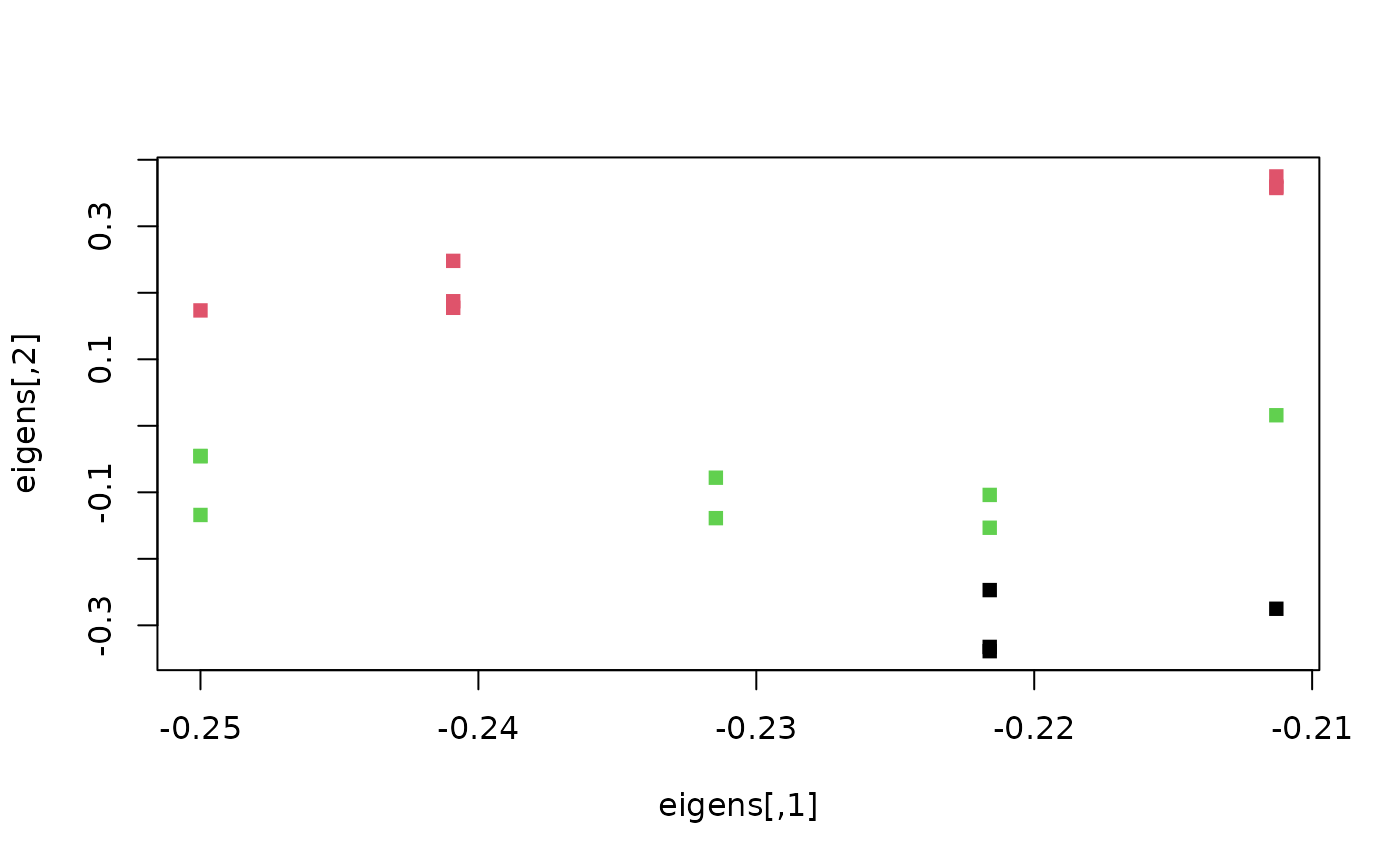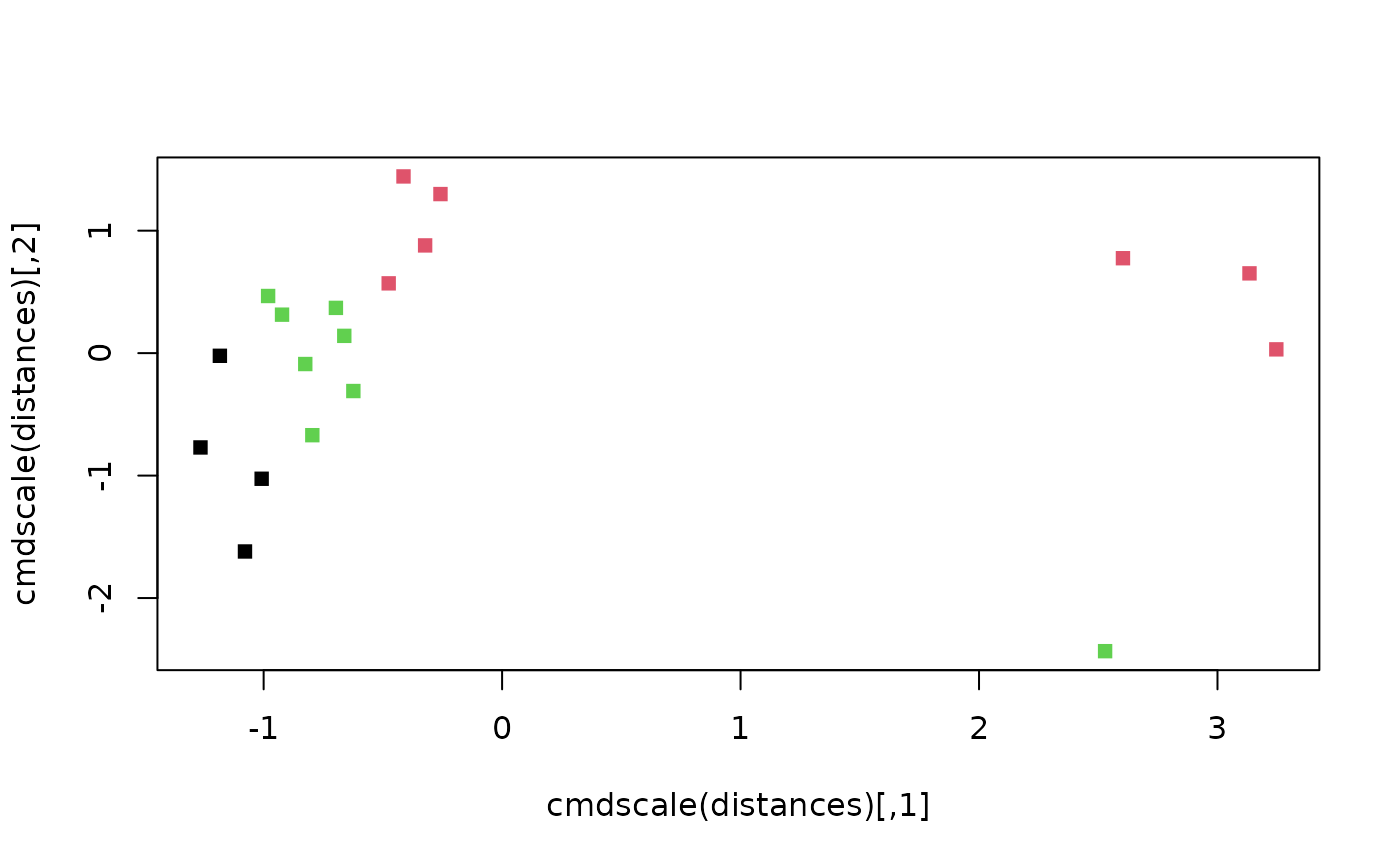Spectral clustering emphasizes nearest neighbours when forming clusters; it avoids some of the issues that arise from clustering around means / medoids.

SpectralEigens(D, nn = 10L, nEig = 2L)

SpectralClustering(D, nn = 10L, nEig = 2L)

## Arguments

D

Square matrix or dist object containing Euclidean distances between data points.

nn

Integer specifying number of nearest neighbours to consider

nEig

Integer specifying number of eigenvectors to retain.

## Value

SpectralEigens() returns spectral eigenvalues that can then be clustered using a method of choice.

Other tree space functions: MSTSegments(), MapTrees(), MappingQuality(), median.multiPhylo()

## Author

Adapted by MRS from script by Nura Kawa

## Examples

library('TreeTools', quietly = TRUE, warn.conflict = FALSE)
trees <- as.phylo(0:18, nTip = 8)
distances <- ClusteringInfoDistance(trees)
eigens <- SpectralEigens(distances)
# Perform clustering:
clusts <- kmeans(dist(eigens), centers = 3)
plot(eigens, pch = 15, col = clusts$cluster)plot(cmdscale(distances), pch = 15, col = clusts$cluster)